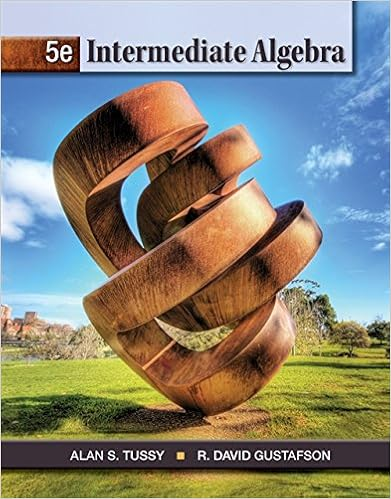hw_1_s16_sol - MAT 243 WRITTEN HOMEWORK 1(1 Fill in the...

• Homework Help
• 2
• 100% (10) 10 out of 10 people found this document helpful

This preview shows page 1 - 2 out of 2 pages.

We have textbook solutions for you!
The document you are viewing contains questions related to this textbook.The document you are viewing contains questions related to this textbook.
Chapter 2 / Exercise 4
Intermediate Algebra
Tussy/GustafsonExpert Verified
MAT 243 WRITTEN HOMEWORK 1 (1) Fill in the blank in the statements below: (a) Two propositions are logically equivalent if and only if they have the same truth values. (b) A tautology is a proposition that is always true (c) The negation of ”if p then q ” is p ∧ ¬ q (d) ” r is a sufficient condition for s ” means if r then s . (e) ” a is a necessary condition for b ” means if b then a . (2) Given the conditional: I go to the beach or I go out dancing, if I am done with my work. (a) State the converse of this statement:
(b) State the contrapositive of this statement:
(c) State the negation of this statement:
(3) Write the following statements in symbolic form. Identify the propositional functions (if needed), universe of discourse you are using:
We have textbook solutions for you!
The document you are viewing contains questions related to this textbook.The document you are viewing contains questions related to this textbook.
Chapter 2 / Exercise 4
Intermediate Algebra
Tussy/GustafsonExpert Verified
•••